# Set Operations

MathematicsComputer EngineeringMCA

#### Mathematics for Data Science and Machine Learning using R

64 Lectures 10.5 hours

#### Engineering Mathematics - Numerical Analysis & more

6 Lectures 1 hours

#### Advanced Mathematics Preparation for JEE/CET/CAT

30 Lectures 3.5 hours

Venn diagram, invented in 1880 by John Venn, is a schematic diagram that shows all possible logical relations between different mathematical sets.

Examples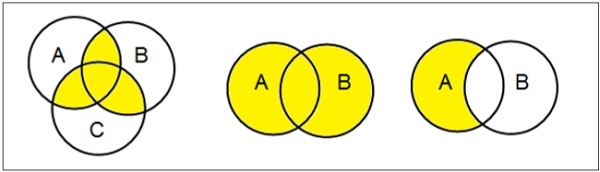## Set Operations

Set Operations include Set Union, Set Intersection, Set Difference, Complement of Set, and Cartesian Product.

### Set Union

The union of sets A and B (denoted by A ∪ B) is the set of elements that are in A, in B, or in both A and B. Hence, A ∪ B = { x | x ∈ A OR x ∈ B }.

Example − If A = { 10, 11, 12, 13 } and B = { 13, 14, 15 }, then A ∪ B = { 10, 11, 12, 13, 14, 15 }. (The common element occurs only once)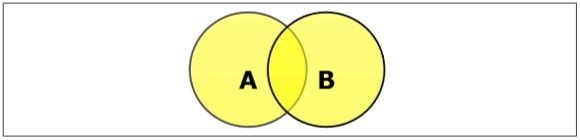### Set Intersection

The intersection of sets A and B (denoted by A ∩ B) is the set of elements which are in both A and B. Hence, A ∩ B = { x | x ∈ A AND x ∈ B }.

Example − If A = { 11, 12, 13 } and B = { 13, 14, 15 }, then A ∩ B = { 13 }.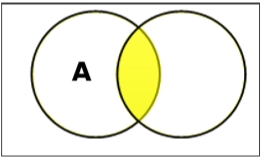### Set Difference/ Relative Complement

The set difference of sets A and B (denoted by A – B) is the set of elements that are only in A but not in B. Hence, A - B = { x | x ∈ A AND x ∉ B }.

Example − If A = { 10, 11, 12, 13 } and B = { 13, 14, 15 }, then (A - B) = { 10, 11, 12 } and (B - A) = { 14, 15 }. Here, we can see (A - B) ≠ (B - A)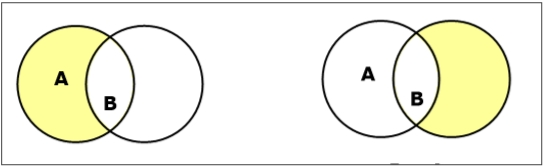### Complement of a Set

The complement of a set A (denoted by A’) is the set of elements which are not in set A. Hence, A' = { x | x ∉ A }.

More specifically, A'= (U - A) where U is a universal set that contains all objects.

Example − If A = { x | x belongs to set of odd integers } then A' = { y | y does not belong to set of odd integers }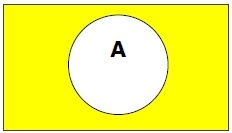### Cartesian Product / Cross Product

The Cartesian product of n number of sets A1, A2, ... An denoted as A1 × A2 ... × An can be defined as all possible ordered pairs (x1, x2, ... xn) where x1 ∈ A1, x2 ∈ A2, ... xn ∈ A_n

Example − If we take two sets A = { a, b } and B = { 1, 2 },

The Cartesian product of A and B is written as − A × B = { (a, 1), (a, 2), (b, 1), (b, 2)}

The Cartesian product of B and A is written as − B × A = { (1, a), (1, b), (2, a), (2, b)}